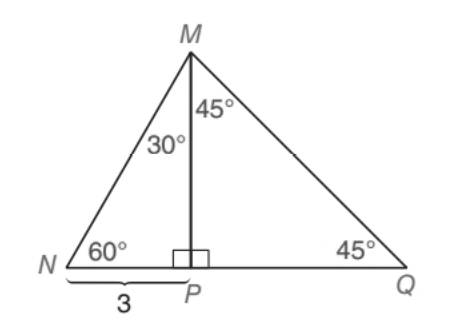Chapter 5.5, Problem 20EElementary Geometry For College St...

7th Edition
Alexander + 2 others
ISBN: 9781337614085

Solutions

Chapter
SectionElementary Geometry For College St...

7th Edition
Alexander + 2 others
ISBN: 9781337614085
Textbook Problem

Given: ∆ N Q M with anglesas shown in thedrawing M P ¯ ⊥ N Q ¯ Find: N M ,   M P ,   M Q , P Q , and NQTo determine

To find:

NM, MP, MQ, PQ, and NQ in NQM with angles as shown in the drawing and with

MP¯NQ¯

Explanation

Approach:

For a right triangle, for which the measure of the interior angles 30°, 60°, and 90°; if ‘a’ is the length of measure of the shorter leg; opposite to the angle 30°, then the length of the other two sides is given by

Length of the longer leg (opposite to 60°) =a3

Length of the hypotenuse (opposite to 90°) =2a.

In general

Length of the longer leg =3× (Length of the shorter leg)

Length of the hypotenuse =2× (Length of the shorter leg)

For a right triangle for which the measure of the interior angles 45°, 45°, and 90°, if ‘a’ is the length of measure of one of the leg; opposite to the angle 45°, then the length of the other two sides is given by

Length of the other leg =a

Length of the hypotenuse =a2

In general

Length of the legs are equal.

Length of the hypotenuse =2× (Length of one of the legs)

Calculation:

Given,

MPNQ

Thus, NQM is divided into two right triangles as MNP and MPQ.

Here, MNP is of 30°-60°-90° type of right and MPQ is of 45°-45°-90° type of right triangle.

Now, in MNP NM is the hypotenuse and MP is the leg opposite to the 60° angle and which is the longer leg and the leg NP is the shorter leg.

Thus, in MNP

MN= Hypotenuse,

MP= Longer leg, and

NP= Shorter leg =3 units.

30°-60°-90° theorem.

In a right triangle whose angle measure 30°, 60°, and 90°, the hypotenuse has a length equal to twice the length of the shorter leg, and the longer leg is the product of 3 and the length of the shorter leg.

The hypotenuse =2× (Length of shorter leg)

NM=2×NP

NM=2×3

NM=6 units.

Again, by 30°-60°-90° theorem, we have

The longer leg =3× (Length of shorter leg)

MP=3×(NP)

MP=3×(3)

Mp=33 units

MP=5

Still sussing out bartleby?

Check out a sample textbook solution.

See a sample solution

The Solution to Your Study Problems

Bartleby provides explanations to thousands of textbook problems written by our experts, many with advanced degrees!

Get Started

Differentiate the function. H(u) = (3u 1)(u + 2)

Single Variable Calculus: Early Transcendentals, Volume I

True or False: The graph at the right is the graph of a function.

Study Guide for Stewart's Single Variable Calculus: Early Transcendentals, 8th

Find for defined implicity by .

Study Guide for Stewart's Multivariable Calculus, 8th